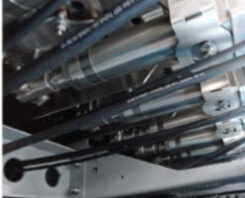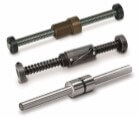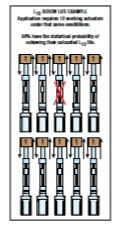# “How long will this actuator last?”

By Tolomatic on June 1, 2016“How long will it last?” Every machine design engineer gets asked this question and will have to calculate the anticipated life of the machine – life that’s based on machine components including linear actuators. Engineers also have to consider expected life when they’re evaluating competing components.

Calculating linear actuator life can be straight-forward for ball screw and roller screw actuators using the L10 life formula for ball bearings.  The life calculation focuses on these rolling elements because the screw/nut combination is an actuator’s critical moving component. Our newest guide explains all the calculations.  Get your copy here.

## DefinitionsDynamic Load Rating (DLR): This is a number that represents a constant load under which a ball bearing device will achieve 1,000,000 revolutions (rotations) of rated life. It is usually provided by the manufacturer and represented by the letter C.

Constant load:  This is a load that remains unchanged along the full working cycle.

Equivalent Dynamic Load:  When an application has a varying load, you have to calculate the equivalent dynamic load or Pe. Equivalent dynamic load is a dynamic load acting on the screw which, if applied constantly, would have the same effect on screw life as the combined actual loads.In the case of constant load, equivalent load = actual load

L10 (or B10) Life: L10 is a calculation of life at which 10% of bearings in the same application can be expected to fail due to classic fatigue failure. L10 life for a group of identical screw actuators operating under the same conditions is the number of revolutions (or distance of travel) which 90% of these actuators have the statistical probability to achieve.

This calculation provides a theoretical life estimate based on a collection of statistics. This is not a guarantee of performance but a guide for expected life.

## How to calculate L10 life with a constant load

To estimate life with a constant load, the underlying formula is:

L10 = (C/Pe)3 x l

C = Dynamic load rating (lbf) or (N)

Pe = Equivalent load (lbf) or (N

l = Screw lead (in/rev) or (mm/rev)

For example, in a case where:

C = 10,000 lbf

Pe = 5,000 lbf

l = 5 mm

L10 = (10,000/5,000)3 x 5 =

(2)3 x 5 =

8 x 5=

40 =

40 million mm

## How to calculate L10 life with a varying load

When a load varies during the working cycle you first have to calculate the equivalent dynamic load (Pe). Here’s the formula:

Pe = 3 √{ [L1(P1)3+L2(P2 )3+L3(P3 )3+Ln(Pn )3]/L}

Pe = Equivalent load (lbs) or (N)

Pn = Each increment at different load (lbs) or (N)

L = Total distance traveled per cycle (extend + retract stroke) (L = L1 + L2 + L3 + Ln)

Ln = Each increment of stroke (in) or (mm) at different load

Then you can use the calculated Pe in the life calculation formula:

L10 = (C/Pe)3 x l

See our new guide for examples of how to use this formula. And watch for a future issue of this blog where we’ll explain how to compare the L10 life of two actuators and how to estimate life in units of time (days, years) rather than distance.